## Screenshots

••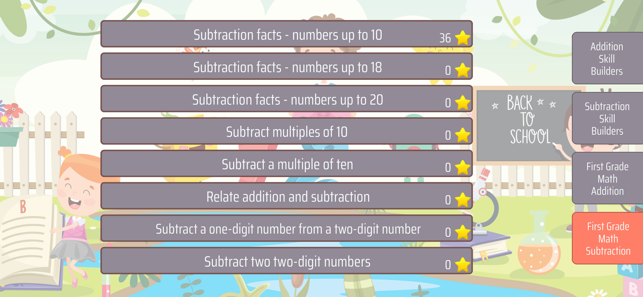•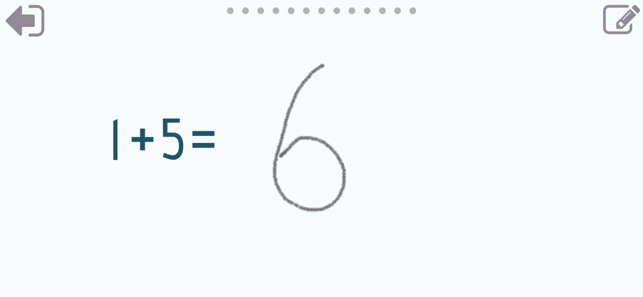•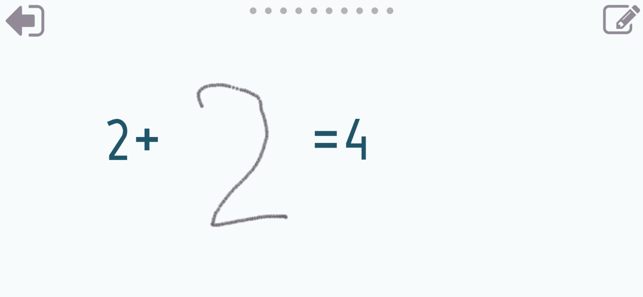•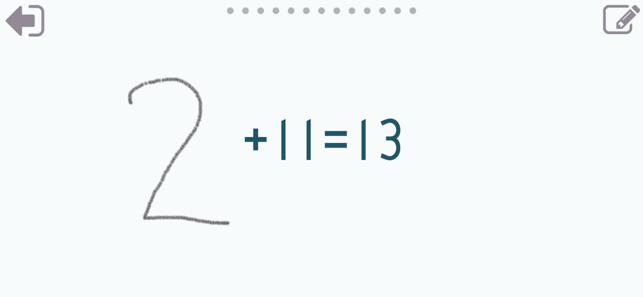•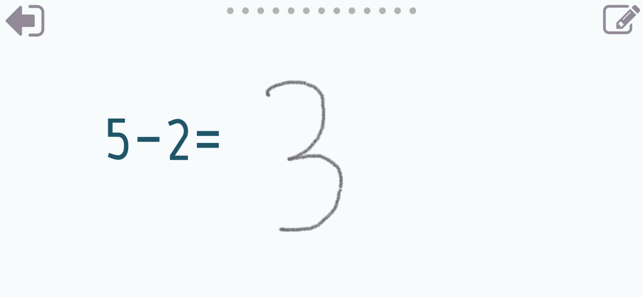•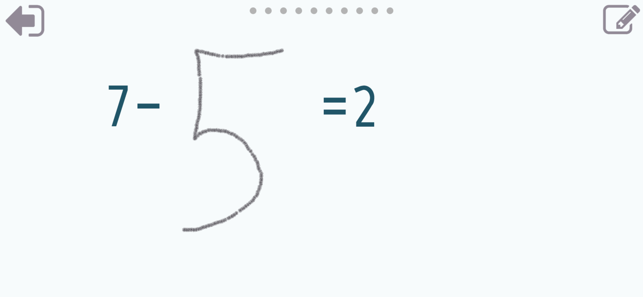•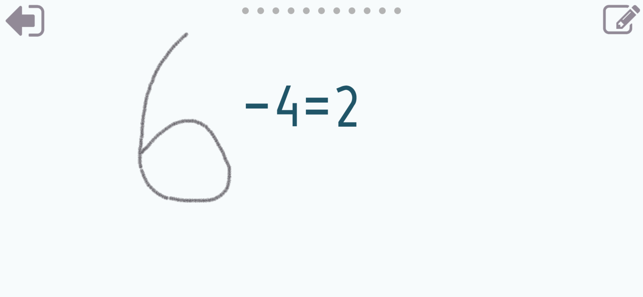•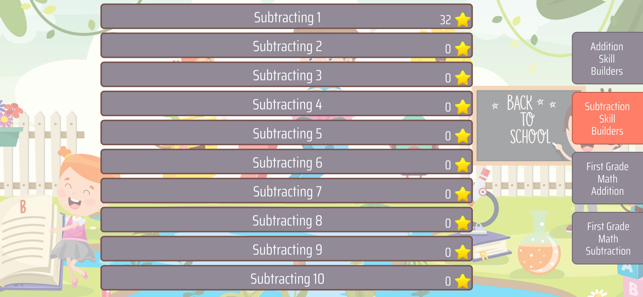•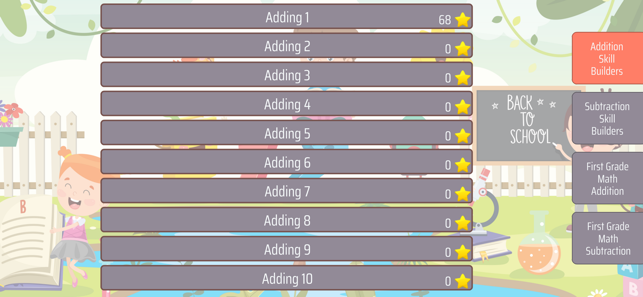## Description

With Addition Skill Builders you can practice and improve the following skills:

With Subtraction Skill Builders you can practice and improve the following skills:
- Subtracting 1
- Subtracting 2
- Subtracting 3
- Subtracting 4
- Subtracting 5
- Subtracting 6
- Subtracting 7
- Subtracting 8
- Subtracting 9
- Subtracting 10

With First grade Math Addition you can practice and improve the following skills:
- Add a number to a multiple of ten
- Add two multiples of ten
- Add three numbers up to 10 each

With First grade Math Subtraction you can practice and improve the following skills:
- Subtraction facts - numbers up to 10
- Subtraction facts - numbers up to 18
- Subtraction facts - numbers up to 20
- Subtract multiples of 10
- Subtract a multiple of ten
- Subtract one-digit number from two-digit number
- Subtract two two-digit numbers

Choose between two different modes:
- Math Trainer
- Math Shot mini-game

Math Trainer mode helps you to master the math facts, you can solve math problems at you own pace without any time constrain.

Math Shot is a math mini-game with fun and engaging game-play and as we all know learning through play and fun is more effective. The game difficulty adapts to player's skills and makes the game suitable for all ages. Math Shot helps students to develop math fact fluency - the result of consistent learning, repetition, and practice.

Version 2.0.2

Bugfixes.

## Information

Provider
Sergey Malugin
Size
33.5 MB
Category
Education
Compatibility

Requires iOS 8.0 or later. Compatible with iPhone, iPad and iPod touch.

Languages

English

Age Rating
4+
Price
S\$ 1.48

## Supports

•